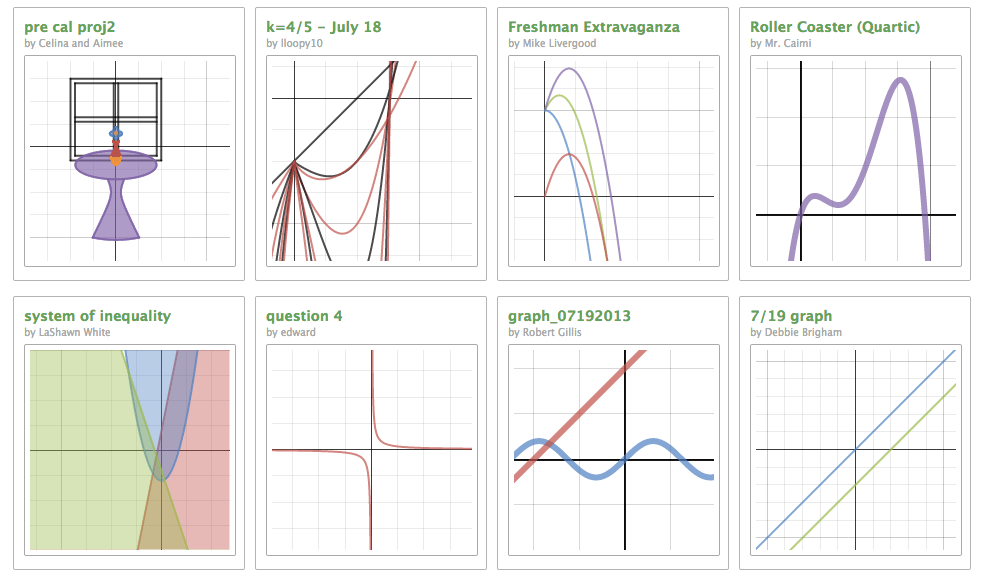# Free Web-based Tools for Math Visualization

Mathematics is known to be a beautiful yet very abstract subject. It may be easy for mathematician to visualize complicate equations and functions in their brain, but sometimes can be challenging for students or starters to do this. However, with the advance of computer and graphing technologies. In the following i would like to introduce a few cool tools that are free for math visualization.

The first tool that I like is Wolfgram Alpha. It is a very cool “Computational Knowledge Engine” (that’s what they call themselves). You can type in questions you want to know from basic algebra to a country’s population. Here I typed in the question of “how many people are there in China” since I am from there. They it will search and collect all information about China’s population and then generate charts based on information it collects:Ok I know, we are talking about MATH visualization. So here it is:  if you type in a equation or a problem, Wolfgram gives you the chart based this. For example, typing in: plot r=cos(3*theta/5), you will get this:Or, it will solve equations for you: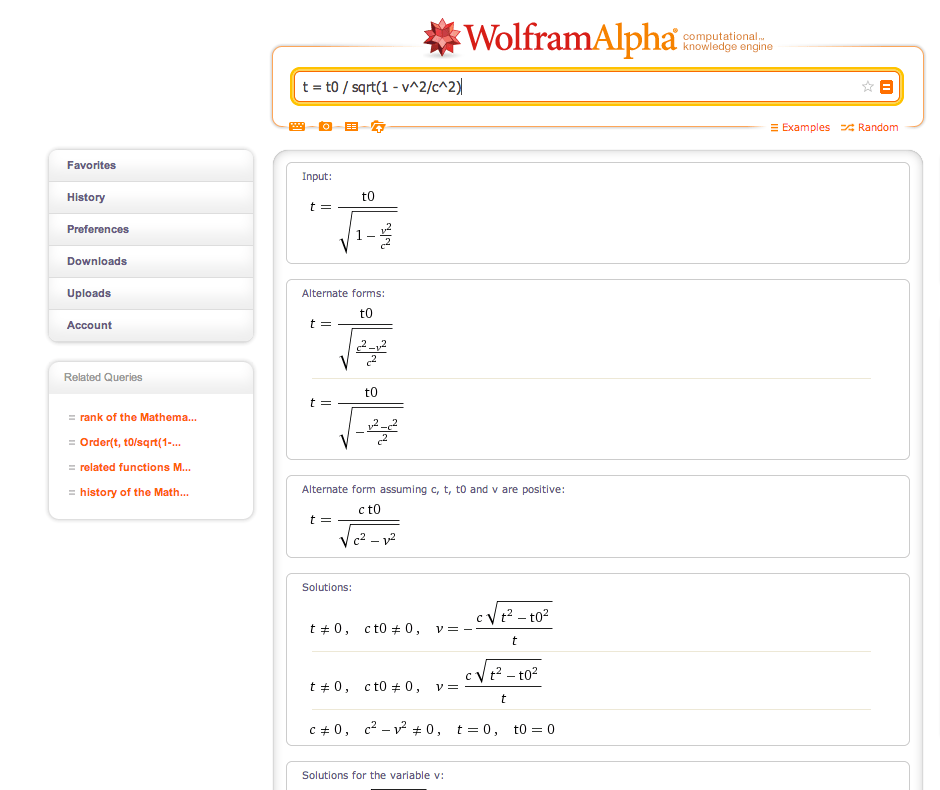# Wolfram Education Portal

Wolfram also provides an educational portal. The portal is currently in Beta version (as of July 2013), and you will need to create an account in order to use it. The content of Wolfram Educational Portal is based on the open book of CK-12 Algebra and it covers but not limited to topics such as equations and functions, real numbers, equations of lines, graphs of equations and functions, solving systems of equations, and polynomials. These contents can be demostrated directly on the Wolfram Educational Portal website. Or teachers can download them to computer and then view them in the Computable Document Format (CDF).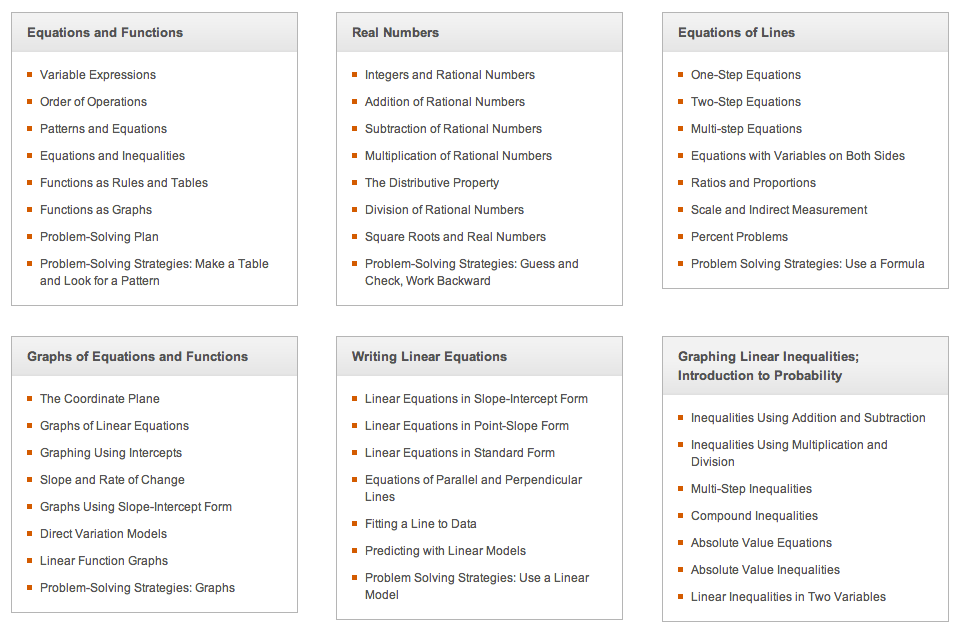The second interesting online math visulization tool is desmos.com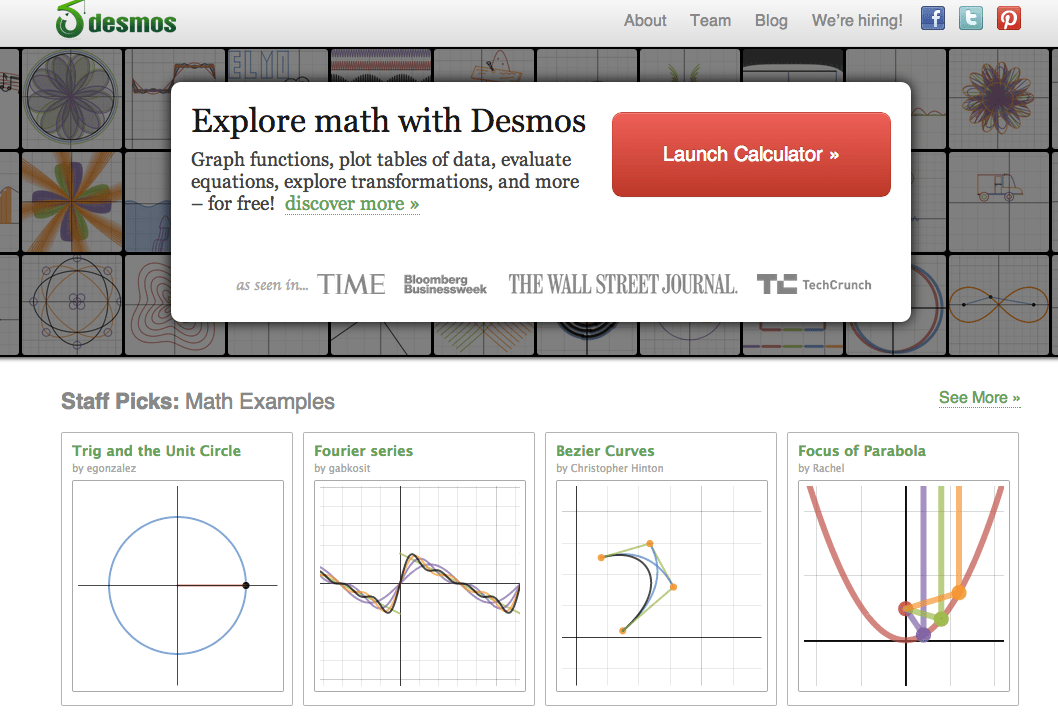Having a similar function of Wolfram Alpha, desmos provides on-screen math equation creation “keyboard” for easy creation of equations.

Desmos is great for graphing at the k-12 level because it has sliders, can handle implicit relations, and can handle inequalities. However Desmos can’t handle calculus, which some high school students take.

Desmos allows multiple equations and plots in the same time, therefore students can compare the change of the plots when the factors of the equations change: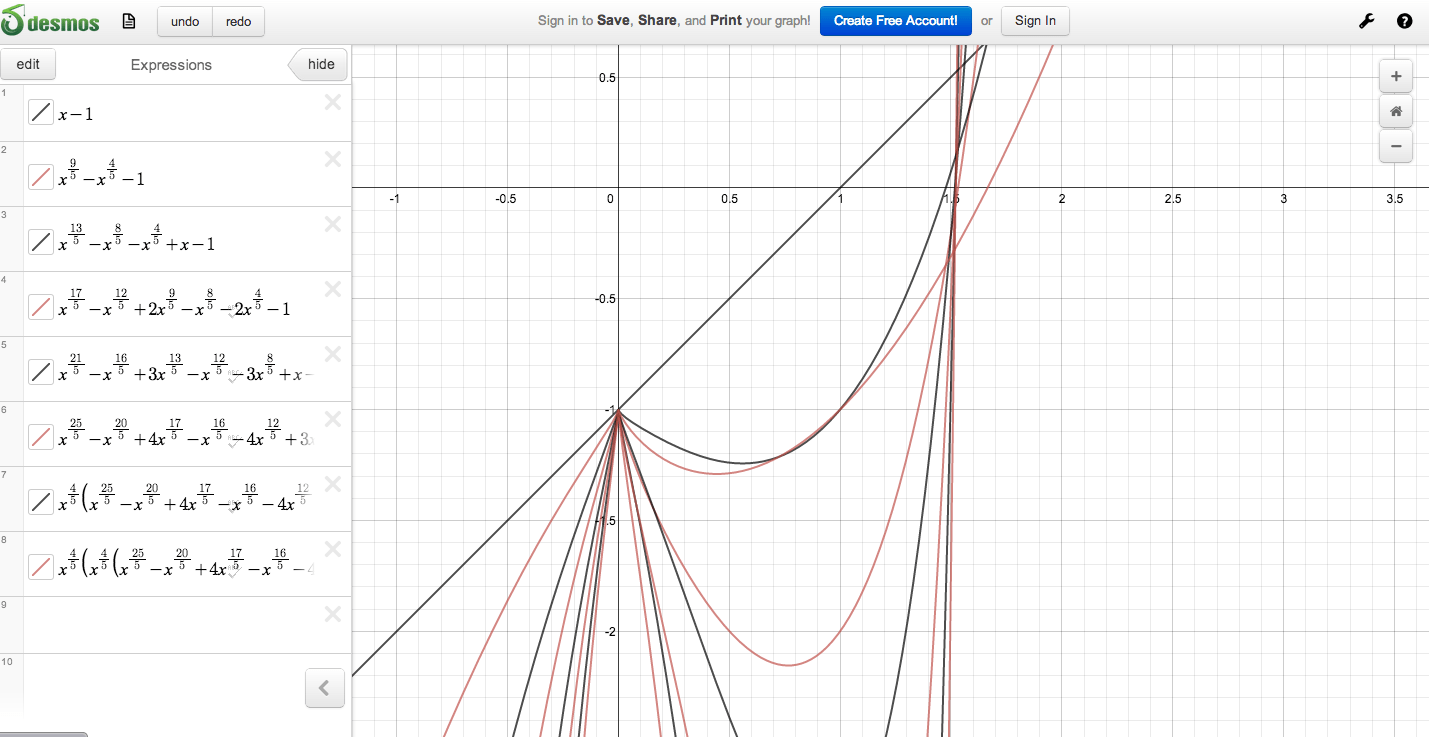Type in an equation, then change the values of each factor, the plot on the right will change: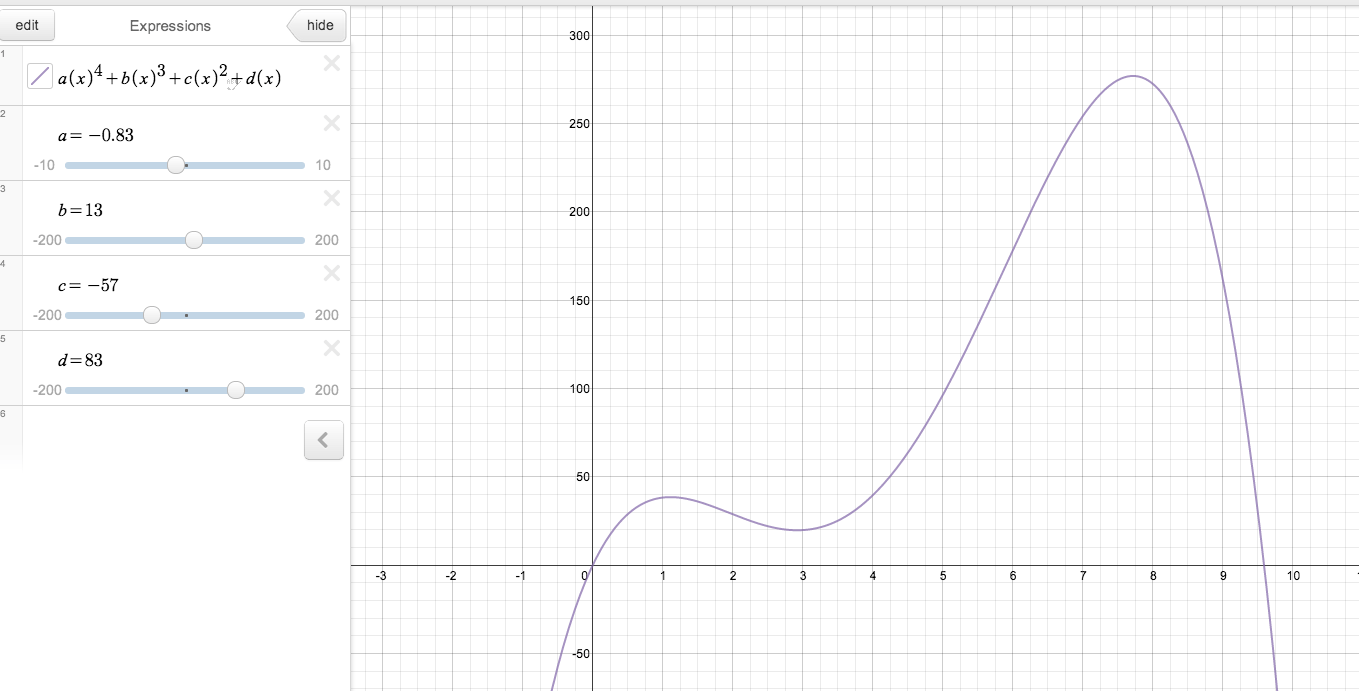The exploration function on Desmos allows students to choose a topic on the left and then interact with the graph or modify the expressions:Lastly, Desmos has a nice gallery of math plots that were saved and shared by its community members, which may be easily adapted to your class: